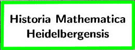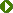#Lazarus Fuchs.

(Immanuel Lazarus Fuchs, born in Moschin, near Posen, May 5, 1833, died at Berlin, April 26, 1902.)

Fuchs is dead. This announcement must have caused deep sorrow in the heart of many American mathematicians. For many of us have been his pupils, and to some of us his example has been the greatest inspiration of our lives. The writer of this little sketch is one of these. He remembers how he looked forward to the time when he would be fitted to attend Fuchs's lectures. He remembers the small and crowded lecture-room in the University of Berlin, poorly ventilated, stuffy and hot in the summer days, but so full of meaning and inspiration to the earnest and thoughtful student. Fuchs was not a brilliant lecturer. He spoke in a quiet, undemonstrative manner, but what he said was full of substance. To the student there was the inspiration of seeing a mathematical mind of the highest order full at work. For Fuchs worked when he lectured. He was rarely well prepared, but produced on the spot what he wished to say. Occasionally he would get lost in a complicated computation. Then he would look around at the audience over his glasses with a most winning and child-like smile. He was always certain of the essential points of his argument, but numerical examples gave him' a great deal of trouble. He was fully conscious of this failing, and I remember well one occasion when, after a lengthy discussion, he laid considerable emphasis upon the fact that " in this case, two times two is four."

The mathematical papers of Fuchs are very numerous, but excepting a few of his earliest attempts, they are all connected directly or indirectly with the theory of linear differential equations. This was the province which, to quote the words of Auwers when he introduced Fuchs to the Berlin Academy of Sciences, he had added to the mathematical kingdom. Of course the conquest of this new territory had been prepared by others. The theory of functions, which was an essential prerequisite had been built up by Cauchy and Riemann. The work of Briot and Bouquet on differential equations of the first order was an illustration of the application of this new theory to the treatment of differential equations. But to Fuchs belongs the credit of first applying the theory of functions to the linear differential equations of any order, with rational coefficients. His paper in the sixty-sixth volume of Crelles Journal is a classic, and to this day I know of no clearer exposition of the fundamental principles involved. It is true that Riemaim was acquainted with these principles, as his posthumous paper on this subject proves. It is true also that Fuchs took his immediate inspiration from Riemann's famous paper on the hypergeometric series. But all of this does not lessen the credit due to Fuchs. To generalize is one of the functions of the mathematician. This Fuchs did. Riemann also did this, but his paper was never published until the theory had long been developed by Fuchs. It is interesting to notice in this connection the difference between the points of view adopted by Riemann and Fuchs. Fuchs starts out with the linear differential equation of the nth order whose coefficients are rational functions of x. By a rigorous examination of the convergence of the series, which formally satisfies the differential equation he finds that these equations have a very important and characteristic property. The singular points of the solutions are fixed, i. e., they are independent of the constants of integration and can be found without first integrating the differential equation. They are in fact included among the poles of its coefficients. He then shows that a fundamental system of solutions undergoes a linear substitution with constant coefficients when the variable x describes a circuit enclosing such a singular point, and from this behavior of the solutions derives expressions for them, valid in a circular region surrounding that point and reaching as far as the next singular point. He thus establishes the existence of systems of n functions, uniform, finite and continuous, except in the vicinity of certain points, and undergoing linear substitutions with constant coefficients when the variable x describes circuits around these points.

Riemann's point of view was exactly opposite to this. With him it was a matter of principle not to define a function by an expression, but by a characteristic property. He, therefore, starts out with the assumption of a system of n functions uniform, finite and continuous, except in the vicinity of certain arbitrarily assigned points, and undergoing an arbitrarily assigned linear substitution when the variable x describes a closed path around such a point. He then shows that such a system of functions will satisfy a linear differential equation of the nth order. But the theorem that the branch points and the substitutions may be arbitrarily assigned, ought to be proved at the outset if this point of view is to be adopted. Much has since been done on this question, which is really a fundamental one in the theory of linear differential equations.

This is not the place to go into details. Suffice it to say that the theory of linear differential equations was placed by Fuchs upon a solid and adequate foundation. He and his followers have reared upon this a noble structure. He himself characterized a class of such equations, called after him Fuchsian, whose solutions are everywhere regular. He studied the question of algebraic integrability which has so many points of contact with other questions of importance. He studied by his own methods the periods of an elliptic integral as function of the modulus, for Legendre had shown that these verify a linear differential equation of the second order. By considering the modulus inversely as a function of the quotient of the periods, a uniform automorphic function, now known as the modular function, was obtained. The theory of modular functions, and more generally of automorphic functions owes much to Fuchs, as well as to Klein and to Poincaré, who as an indication of this fact has named a large class of such functions Fuchsian. Fuchs has introduced other transcendental functions into analysis, connected with a linear differential equation in much the same way as the abelian functions are connected with an algebraic equation. Little has yet been done with these beyond the proof of their existence.

We have already mentioned that one of the first results obtained by Fuchs in his classical memoir was the fact that the branch points of a linear differential equation are fixed. Fuchs himself was the first to ask the question; are there other equations of this kind ? In a beautiful paper in the Sitzungsberichte der Berliner Akademie, he started the investigation of this important question, confining himself, however, to differential equations of the first order. Poincaré completed the investigation in a remarkable manner, the result being that no essentially new functions could be defined by differential equations of the first order with fixed singular points. Painlevé has since then found that transcendental functions essentially new can be defined by such equations of order higher than the first.

We will close this brief sketch by translating a sentence, which is as characteristic of the modern theory of differential equations, as the famous definition of Kirchhoff is of modern mechanics. Fuchs says in his famous paper in Crelle's Journal: " In the present condition of science it is not so much the problem of the theory of differential equations to reduce a given differential equation to quadratures, as to deduce from the equation itself the behavior of its integrals at all points of the plane, i. e., for all values of the complex variable."

This is the present point of view in the theory of differential equations. The first chapter of this theory, that of linear differential equations, has been far advanced, although not completed, by Fuchs and his pupils. Something has been done on later chapters, but not much. The theory of non-linear differential equations is one of the central problems of modern mathematics, but it has not yet found its Fuchs.

E. J. WlLCZYNSKI.

University of California
Berkeley, July 8, 1902.

aus:
Bulletin of the American Mathematical Society. - N.S. 9.1902/03 (1903). - S. 46-49
Signatur UB Heidelberg: L 21-1-4::2:9

Ernest Julius Wilczynki, * 13. November 1876 in Hamburg, † 14. September 1932 in Denver (Colorado), studierte in Berlin und schloss das Studium 1897 mit der Promotion ab.

Letzte Änderung: Mai 2014     Gabriele DörflingerKontakt

Zur InhaltsübersichtHistoria MathematicaHomo HeidelbergensisLazarus Fuchs